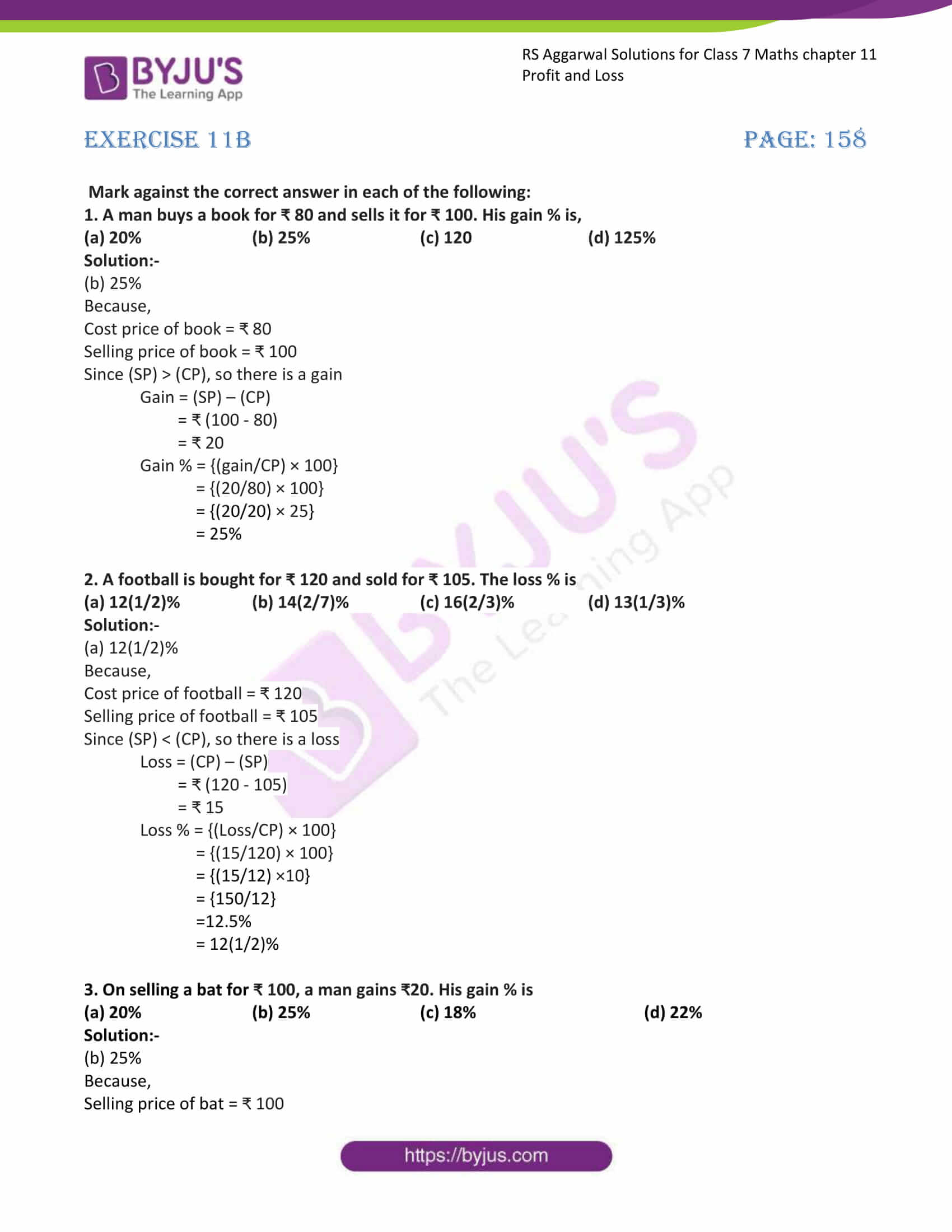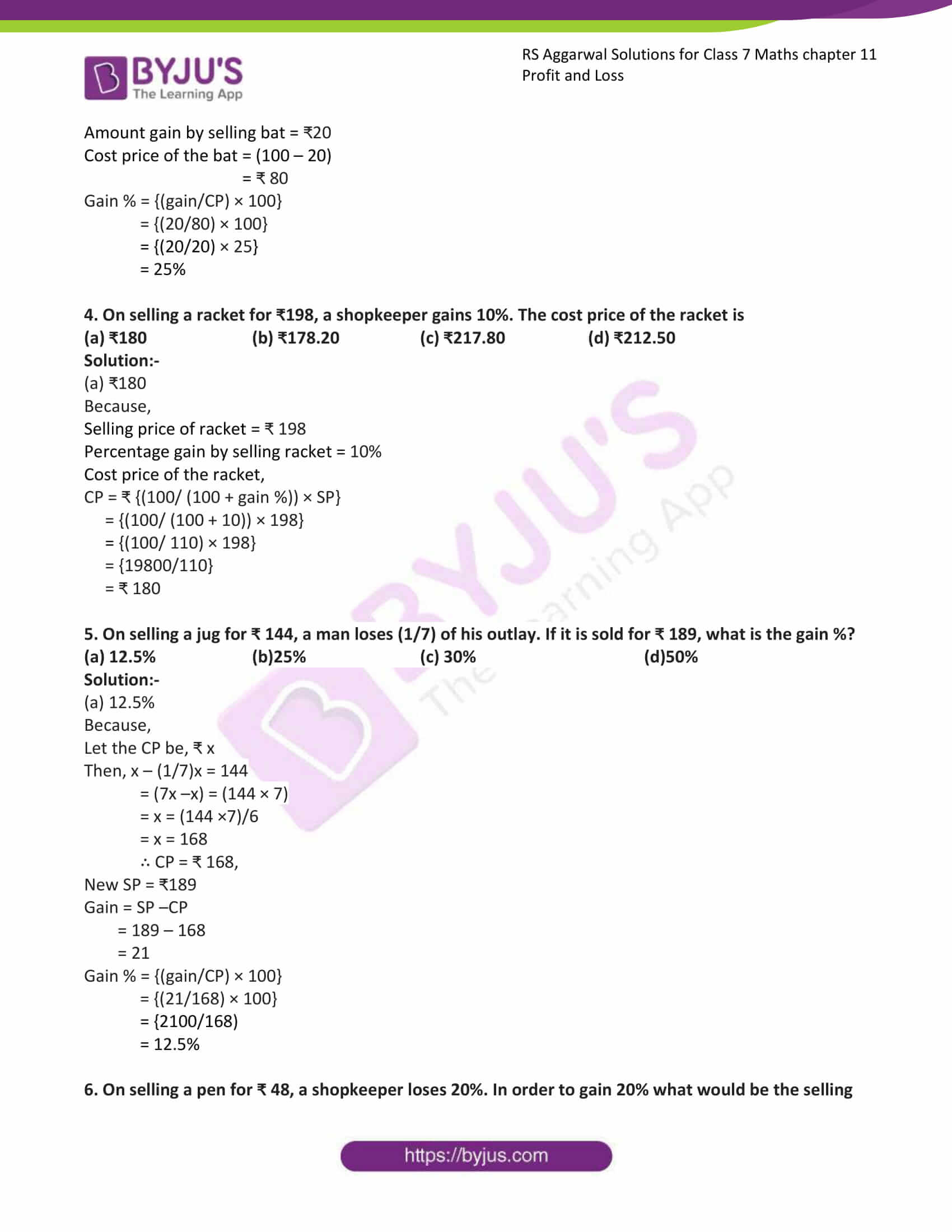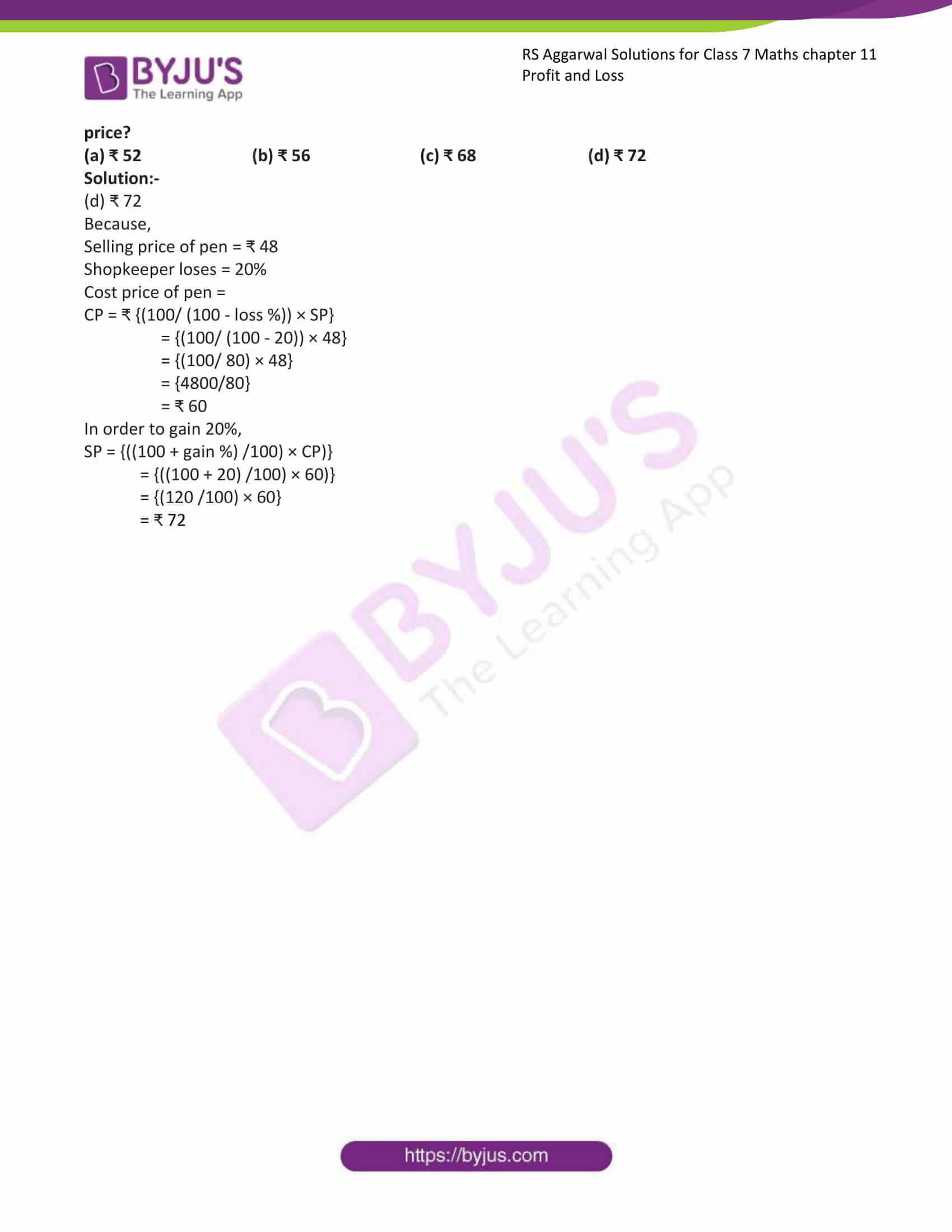# RS Aggarwal Solutions for Class 7 Maths Exercise 11B Chapter 11 Profit and loss

RS Aggarwal Solutions for Class 7 Maths Exercise 11B Chapter 3 Profit and Loss in simple PDF are available here. This exercise of RS Aggarwal Solutions for Class 7 Chapter 11 contains objective type questions, and it covers all the topics in the chapter with different types of questions. Students who go through these questions can be able to solve any problems. Students are suggested to try solving the questions from RS Aggarwal Solutions for Class 7 Maths Chapter 11 Profit and loss.

## Download the PDF of RS Aggarwal Solutions For Class 7 Maths Chapter 11 Profit and Loss – Exercise 11B### Access answers to Maths RS Aggarwal Solutions for Class 7 Chapter 11 – Profit and Loss Exercise 11B

Mark against the correct answer in each of the following:

1. A man buys a book for ₹ 80 and sells it for ₹ 100. His gain % is,

(a) 20% (b) 25% (c) 120 (d) 125%

Solution:-

(b) 25%

Because,

Cost price of book = ₹ 80

Selling price of book = ₹ 100

Since (SP) > (CP), so there is a gain

Gain = (SP) – (CP)

= ₹ (100 – 80)

= ₹ 20

Gain % = {(gain/CP) × 100}

= {(20/80) × 100}

= {(20/20) × 25}

= 25%

2. A football is bought for ₹ 120 and sold for ₹ 105. The loss % is

(a) 12(1/2)% (b) 14(2/7)% (c) 16(2/3)% (d) 13(1/3)%

Solution:-

(a) 12(1/2)%

Because,

Cost price of football = ₹ 120

Selling price of football = ₹ 105

Since (SP) < (CP), so there is a loss

Loss = (CP) – (SP)

= ₹ (120 – 105)

= ₹ 15

Loss % = {(Loss/CP) × 100}

= {(15/120) × 100}

= {(15/12) ×10}

= {150/12}

=12.5%

= 12(1/2)%

3. On selling a bat for ₹ 100, a man gains ₹20. His gain % is

(a) 20% (b) 25% (c) 18% (d) 22%

Solution:-

(b) 25%

Because,

Selling price of bat = ₹ 100

Amount gain by selling bat = ₹20

Cost price of the bat = (100 – 20)

= ₹ 80

Gain % = {(gain/CP) × 100}

= {(20/80) × 100}

= {(20/20) × 25}

= 25%

4. On selling a racket for ₹198, a shopkeeper gains 10%. The cost price of the racket is

(a) ₹180 (b) ₹178.20 (c) ₹217.80 (d) ₹212.50

Solution:-

(a) ₹180

Because,

Selling price of racket = ₹ 198

Percentage gain by selling racket = 10%

Cost price of the racket,

CP = ₹ {(100/ (100 + gain %)) × SP}

= {(100/ (100 + 10)) × 198}

= {(100/ 110) × 198}

= {19800/110}

= ₹ 180

5. On selling a jug for ₹ 144, a man loses (1/7) of his outlay. If it is sold for ₹ 189, what is the gain %?

(a) 12.5% (b)25% (c) 30% (d)50%

Solution:-

(a) 12.5%

Because,

Let the CP be, ₹ x

Then, x – (1/7)x = 144

= (7x –x) = (144 × 7)

= x = (144 ×7)/6

= x = 168

∴ CP = ₹ 168,

New SP = ₹189

Gain = SP –CP

= 189 – 168

= 21

Gain % = {(gain/CP) × 100}

= {(21/168) × 100}

= {2100/168)

= 12.5%

6. On selling a pen for ₹ 48, a shopkeeper loses 20%. In order to gain 20% what would be the selling price?

(a) ₹ 52 (b) ₹ 56 (c) ₹ 68 (d) ₹ 72

Solution:-

(d) ₹ 72

Because,

Selling price of pen = ₹ 48

Shopkeeper loses = 20%

Cost price of pen =

CP = ₹ {(100/ (100 – loss %)) × SP}

= {(100/ (100 – 20)) × 48}

= {(100/ 80) × 48}

= {4800/80}

= ₹ 60

In order to gain 20%,

SP = {((100 + gain %) /100) × CP)}

= {((100 + 20) /100) × 60)}

= {(120 /100) × 60}

= ₹ 72

### Access other exercises of RS Aggarwal Solutions For Class 7 Chapter 11 – Profit and Loss

Exercise 11A Solutions